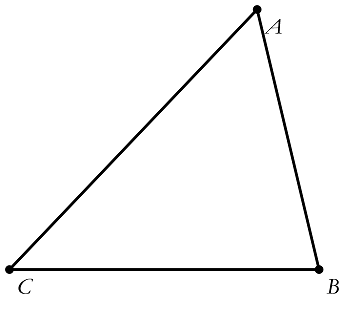# Two Wrongs Make A Right?Nihar and Andrew are trying to find the area of $\triangle ABC$ using the formula

$\dfrac{1}{2} \times \text{base} \times \text{height}.$

Nihar mistakenly multiplies base $AB$ by the height from $A$ $($instead of $C).$ He gets a value of 14.

Andrew mistakenly multiplies base $BC$ by the height from $C$ $($instead of $A).$ He gets a value of 56.

Find the actual area of triangle $\triangle ABC$.

×